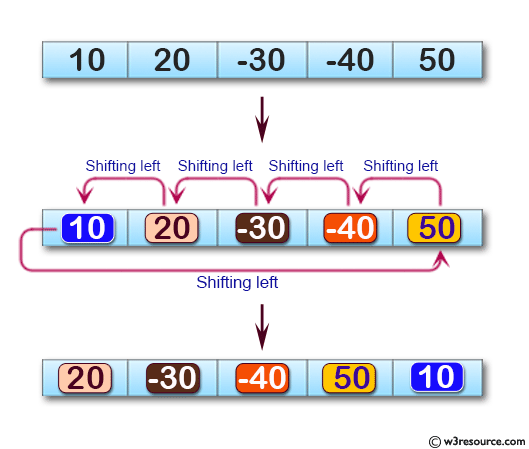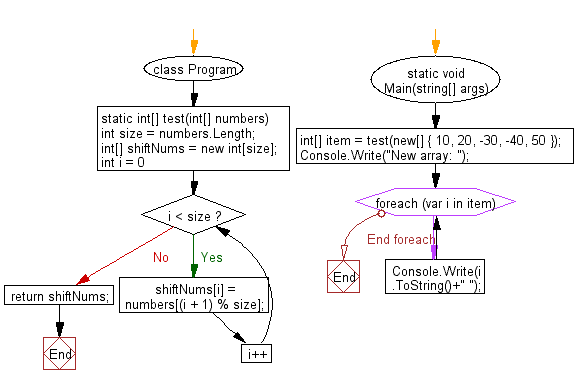﻿ C# - Shift an element in left direction and get a new array# C# Sharp Basic Algorithm Exercises: Shift an element in left direction and return a new array

## C# Sharp Basic Algorithm: Exercise-127 with Solution

Write a C# Sharp program to shift an element in left direction and return a new array.

Pictorial Presentation:Sample Solution:

C# Sharp Code:

``````using System;
namespace exercises
{
class Program
{
static void Main(string[] args)
{
int[] item = test(new[] { 10, 20, -30, -40, 50 });
Console.Write("New array: ");
foreach(var i in item)
{
Console.Write(i.ToString()+" ");
}
}
static int[] test(int[] numbers)
{
int size = numbers.Length;
int[] shiftNums = new int[size];

for (int i = 0; i < size; i++)
{
shiftNums[i] = numbers[(i + 1) % size];
}
return shiftNums;
}
}
}
```
```

Sample Output:

```New array: 20 -30 -40 50 10
```

Flowchart:C# Sharp Code Editor:

Improve this sample solution and post your code through Disqus

What is the difficulty level of this exercise?

Test your Programming skills with w3resource's quiz.

﻿